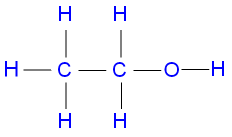# Question #c3e59

May 3, 2017

B. $\text{CH"_3"CH"_2"OH}$ has eight covalent bonds.

#### Explanation:

A. $\text{HOCH"_2"CH"_2"OH}$This compound has 1 $\text{C-C}$ bond, 2 $\text{C-O}$ bonds, 4 $\text{C-H}$ bond, and 2 $\text{O-H}$ bonds, for 9 covalent bonds.

B. $\text{CH"_3"CH"_2"OH}$

EthanolThis compound has 1 $\text{C-C}$ bond, 1 $\text{C-O}$ bond, 5 $\text{C-H}$ bonds, and 1 $\text{O-H}$ bond, for a total of eight covalent bonds.

C. $\text{HOCOCOOH}$This compound contains 1 $\text{C-C}$ bond, 6 $\text{C-O}$ bonds (each $\text{C=O}$ counts as two covalent bonds), and 2 $\text{O-H}$ bonds, for 9 covalent bonds.

D. $\text{HOCH"_2"COOH}$This compound contains 1 $\text{C-C}$ bond, 4 $\text{C-O}$ bonds, 2 $\text{C-H}$ bonds, and 2 $\text{O-H}$ bonds, for 9 covalent bonds.

May 3, 2017

$B$ is the correct option

#### Explanation:

We can use the following formula to determine the number of covalent bonds in a molecule.

$\text{Number of covalent bonds} = \frac{x - y}{2}$

where $x$ is the total number of electrons required to fulfil the octet for atom other than hydrogen atom and doublet for hydrogen atom.

and $y$ is the total number of valence electrons.

A) For compound $H O C {H}_{2} C {H}_{2} O H$

which consists of 2C ,2O and 6H ,we have

$x = 2 \cdot 8 + 2 \cdot 8 + 6 \cdot 2 = 44$

$y = 2 \cdot 4 + 2 \cdot 6 + 6 \cdot 1 = 26$

So $\text{Number of covalent bonds} = \frac{x - y}{2} = \frac{44 - 26}{2} = 9$

B) For compound $C {H}_{3} C {H}_{2} O H$

which consists of 2C ,1O and 6H ,we have

$x = 2 \cdot 8 + 1 \cdot 8 + 6 \cdot 2 = 36$

$y = 2 \cdot 4 + 1 \cdot 6 + 6 \cdot 1 = 20$

So $\text{Number of covalent bonds} = \frac{x - y}{2} = \frac{36 - 20}{2} = 8$

C) For compound $H O C O C O O H$

which consists of 2C ,4O and 2H ,we have

$x = 2 \cdot 8 + 4 \cdot 8 + 2 \cdot 2 = 52$

$y = 2 \cdot 4 + 4 \cdot 6 + 2 \cdot 1 = 34$

So $\text{Number of covalent bonds} = \frac{x - y}{2} = \frac{52 - 34}{2} = 9$

D) For compound $H O C {H}_{2} C O O H$

which consists of 2C ,3O and 4H ,we have

$x = 2 \cdot 8 + 3 \cdot 8 + 4 \cdot 2 = 48$

$y = 2 \cdot 4 + 3 \cdot 6 + 4 \cdot 1 = 30$

So $\text{Number of covalent bonds} = \frac{x - y}{2} = \frac{48 - 30}{2} = 9$